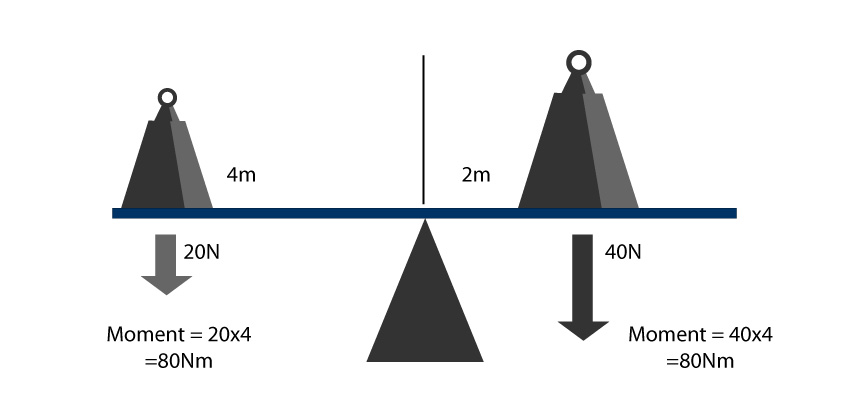## Moments

A Moment is a scientific name for turning forces around a pivot. Forces can make objects turn if there is a pivot. Take a look at the illustration below. The pivot is the point in the middle of it. This pivot makes one end tip-up or down depending on the force applied to one end. This means moments can be equal and opposite if the force applied at both ends are equal and the sea-saw is balanced.

To work out a moment, two things are considered:

• The distance from the pivot that the force is applied.
• The size of the force applied

This is the equation for working out a moment:

##### moment = force × distance

The unit for moment is Nm (newton meter).

Example

If a force of 10 N acted on a see-saw 2 m from the pivot, the moment would be worked as follows:

force × distance = moment

10 × 2 = 20 Nm

Here is an example of balanced moments. 20N at 4m from the pivot is balancing 40N at 2m from the pivot. The objects create moments of 80Nm that are equal and opposite, so the see-saw is balanced.Equal Moments balances out a see-saw

Moments can be useful in many ways. Here are a few examples

• A crowbar uses moments to lift heavy things over a lever.
• You will notice that longer spanners undo nuts a lot easier than shorter spanners. See the illustration below:
• A see-saw will balance if the moments on each side of the pivot are equal. This is why you might have to adjust your position on a see-saw if you are a different weight from the person on the other end.